2019-04-24 09:37:17 whale52hertz 阅读数 86
• ###### C++语音识别开篇

本篇mark老师将教大家使用第三方库的调用来简单的实现语音识别。随着机器学习和人工智能的热闹，国内语音行业也可谓是百花齐放。 语音识别一个伟大的时代已在我们身边悄悄走来。

5918 人正在学习 去看看 杨波Mozilla（缩写MF或MoFo）全称Mozilla基金会，是为支持和领导开源的Mozilla项目而设立的一个非营利组织。Mozilla对语音识别的潜力感到兴奋。他们相信这项技术能够并且将会带来一波创新产品和服务的浪潮，并且应该对所有人开放。Mozilla收到了来自全球范围内的2万多人提供的不同的声音。通常，现有的语音识别服务无法理解不同的口音，而且大多数情况，比起女性更善于理解男性的语音——这是语音识别服务接受训练的数据中存在的偏见结果。Mozilla希望说话者的数量和他们的不同的口音能够创造出一个全球性的代表数据集，从而带来更具包容性的技术。

Mozilla认为，技术应该是开放的，所有人都可以访问，包括语音。Mozilla开发这种技术的方法是由设计开放的，他们非常欢迎更多的合作者和贡献者与之一起工作。

## 更多推荐

2018-10-17 17:28:52 SrdLaplace 阅读数 575
• ###### C++语音识别开篇

本篇mark老师将教大家使用第三方库的调用来简单的实现语音识别。随着机器学习和人工智能的热闹，国内语音行业也可谓是百花齐放。 语音识别一个伟大的时代已在我们身边悄悄走来。

5918 人正在学习 去看看 杨波

CTC是一种端到端的语音识别技术，他避免了需要字或者音素级别的标注，只需要句子级别的标注就可以进行训练，感觉非常巧妙，也很符合神经网络浪潮人们的习惯。特别是LSTM+CTC相较于之前的DNN+HMM，LSTM能够更好的捕捉输入中的重要的点（LSTM随着状态数目增加参数呈线性增加，而HMM会平方增加），CTC打破了隐马尔科夫的假设，把整个模型从静态分类变成了序列分类。

## 语音识别模型

$p(l|x)=\sum_{\pi=\in B^{-1}(l)}p(\pi|x)$

## 前向后向算法（Forward-Backward Algorithm）

$\alpha(t,s)$可以递推的用$\alpha(t-1,s)，\alpha(t-1,s-1)$计算。

$\alpha(1,1)=y_b^1$

$\alpha(1,2)=y_{l_1}^1$

$\alpha(1,u)=0,u>2$

$\alpha(t,0)=0$

$\alpha(t,u)=0,u<U'-2(T-t)-1$

$U'$表示序列$2|l_{1:u}|+1$

$\alpha(t,u)=y_{l'_u}^t\sum_{i=f(u)}^u\alpha(t-1,i)$

$f(u)=\{\begin{array}{} u-1 & if~l'_u=blank~or~l'_{u-2}=l'_u\\u-2 & otherwise \end{array}$

$\beta(T,U')=1$

$\beta(T,U'-1)=1$

$\beta(T,u)=0,u<U'-1$

$\beta(t, u)=0,u>2t$

$\beta(t,u)=\sum_{i=u}^{g(u)}\beta(t+1,i)y^{t'+1}_{l^u_t}$

$g(u)=\{\begin{array}{} u+1 & if~l'_u=blank~or~l'_{u+2}=l'_u\\u+2 & otherwise \end{array}$

$ln(a+b)=ln~a+ln(1+e^{ln~b-ln~a})$

## Loss Function

the CTC loss函数$L(S)$被定义为训练集$S$种正确标签的negative log probability：

$L(S)=-ln\prod_{(x,z)\in S}=-\sum_{(x,z)\in S}ln~p(z|x)$

$L(x,z)=-ln~p(z|x)$

$L(S)=\sum_{(x,z)\in S}L(x,z)$

$\frac{\partial L(S)}{\partial w}=\sum_{(x,z)\in S}\frac{\partial L(x,z)}{\partial w}$

$\alpha(t,u)\beta(t,u)=\sum_{\pi_t=z_u,B(\pi)=z}\prod_{t=1}^Ty_{\pi_t}^t=\sum_{\pi_t=z_u,B(\pi)=z}p(\pi|x)$

$p(z|x)=\sum_{u=1}^{|z'|}\alpha(t,u)\beta(t,u)$

$L(x,z)=-ln~\sum_{u=1}^{|z'|}\alpha(t,u)\beta(t,u)$

$\frac{\partial L(x,z)}{\partial y_k^t}=-\frac{\partial ln~p(z|x)}{\partial y_k^t}=-\frac{1}{p(z|x)}\frac{\partial p(z|x)}{\partial y_k^t}$

$\frac{\partial p(z|x)}{\partial y_k^t}=\frac{1}{y_k^t}\sum_{u\in B(z,k)}\alpha(t,u)\beta(t,u)$

$\frac{\partial L(x,z)}{\partial y_k^t}=-\frac{1}{p(z|x)\cdot y_k^t}\sum_{u\in B(z,k)}\alpha(t,u)\beta(t,u)$

$\frac{\partial L(x,z)}{\partial a_k^t}=-\sum_{k'}\frac{\partial L(x,z)}{\partial y_{k'}^t}\frac{\partial y_{k'}^t}{\partial a_k^t}$

$y_k^t=\frac{e^{a_k^t}}{\sum_{k'}e^{a_{k'}^t}}$

$\frac{\partial y_{k'}^t}{\partial a_k^t}=y_{k'}^t\delta_{kk'}-y_{k'}^ty_{k}^t$

$\frac{\partial L(x,z)}{\partial a_k^t}=y_k^t-\frac{1}{p(z|x)}\sum_{u\in B(z,k)}\alpha(t,u)\beta(t,u)$

## decoder

$h(x)=argmax_{l}p(l|x)$

### 最佳路径解码（best path decoding）

$h(x)=B(\pi^*),s.t.,\pi^*=argmax_{\pi}p(\pi|x)$

### 前缀查找解码（prefix search decoding）

$l^*$表示当前找到最好的的序列，$p^*$表示以$p^*$为开头的序列可能性最高，当$p^*$为开头的序列可能性小于表示当前找到最好的的序列$l^*$出现的可能性时停止循环，认为$l^*$就是最有可能的序列。循环过程中，对于最有可能的前缀尝试后面加入每一个可能的字符，然后计算加入这个字符之后新的字符串出现的概率和以新的字符串为前缀的概率，当新的字符串出现的概率大于$l^*$时更新$l^*$，把以新的字符串为前缀的概率大于$l^*$出现的概率的前缀加入带备选集合，循如果发现剩下字符搭配$p^*$做前缀的概率之和小于$l^*$出现的概率，停止循环，节约计算。循环完毕后把旧的$p^*$从备选集合中剔除，再把集合中概率最大的前缀作为$p^*$
$\gamma(p_n,t)$表示序列$p$$t$个输出非blank，$\gamma(p_b,t)$表示序列$p$$t$个输出为blank。

## tensorflow中的CTC

tf.nn.ctc_loss(labels, inputs, sequence_length)


labels: 标签，一个稀疏矩阵
inputs：模型输出的logits

decoded, _ = tf.nn.ctc_beam_search_decoder(logits, sequence_len, merge_repeated=False)
decoded, _ = tf.nn.ctc_greedy_decoder(logit, sequence_len, merge_repeated=False)


logits：模型输出的logits
sequence_len：实际的长度（tensorflow静态图模型，告诉他实际的音频长度多长）
merge_repeated：把连续输出相同的合并，不同于ctc_merge_repeated，ctc_merge_repeated是把中间没有blank的合并。

## 音识别模型小型综述

• 2011-2012年：发现把GMM换成DNN有30%的提升
• 2013年：发现调整激活函数Maxout对数据量较少的情况有所改善，对大数据量不一定有显著帮助
• 2013-2014年：浅层DNN升级为浅层CNN
• 2014年：开始尝试开始做RNN，尤其是LSTM
• 2014年底2015年初：Hinton 的博士后 Alex Graves，把以前做手写体识别的LSTM 加 CTC 的系统应用在了语音识别领域，在 TIMIT 上做了结果，紧接着谷歌认为这个很有前途，就开始把这些技术推广在大数据上面。
• 2015年：研究者发现 CNN 在时间上做卷积和 LSTM 有很多相似之处但各有特点。LSTM 更擅长做整个时间域的信息整合，因为 CNN 有很强的平移的不变性，那么鲁棒性更强
• 2016年：Deep CNN，WaveNet 等深层 CNN

## 实现的乞丐版语音识别模型

model: 三层lstm模型
loss function: ctc loss
feature: 13阶mfcc系数+2阶动态因子（39）

## 参考文献

Graves A, Fernandez S, Gomez F, et al. Connectionist temporal classification: labelling unsegmented sequence data with recurrent neural networks[C] Proceedings of the 23rd international conference on Machine learning. ACM, 2006: 369-376.

• ###### C++语音识别开篇

本篇mark老师将教大家使用第三方库的调用来简单的实现语音识别。随着机器学习和人工智能的热闹，国内语音行业也可谓是百花齐放。 语音识别一个伟大的时代已在我们身边悄悄走来。

5918 人正在学习 去看看 杨波

1. 语音识别流程简介；
2. Kaldi 的部署使用；
3. 如何训练基于中文的 DFSMN 声学模型；
4. 语音特征提取 MFCC 算法源码解读；
5. 语音识别工具对比。2019-04-07 15:37:37 asrgreek 阅读数 22448
• ###### C++语音识别开篇

本篇mark老师将教大家使用第三方库的调用来简单的实现语音识别。随着机器学习和人工智能的热闹，国内语音行业也可谓是百花齐放。 语音识别一个伟大的时代已在我们身边悄悄走来。

5918 人正在学习 去看看 杨波

##### 我们从下面这段音频说起。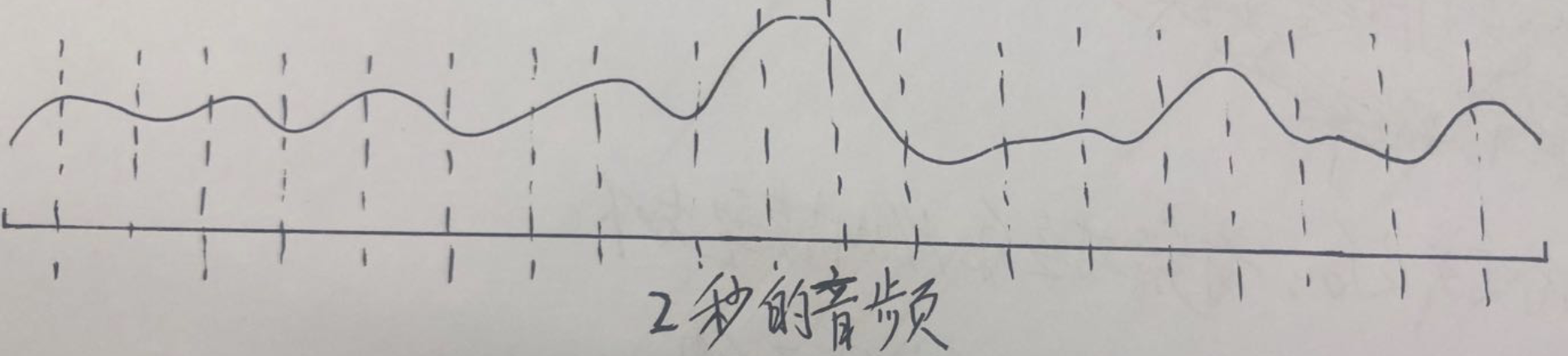###### 一、得到这段音频的标注

1、音素级别的标注。这是训练HMM-GMM模型所需要的标注，也就是说可以直接拿来训练的数据。
2、字节别的标注。实际上我们更多时候拿到是的字级别的标注，因为音素级别的标注工作量很大。但我们可以通过发音词典，将字级别的标注转换成音素级别的标注。这种转换没办法消除多音字的影响，但实际上不会对结果产生太大影响(这一部分可以参考kaldi的compile-train-graphs过程：http://kaldi-asr.org/doc/graph_recipe_train.html)

###### 二、对”n“、”i2“、”h“、”ao3“这4个声韵母分别使用HMM-GMM建模，使用3状态建模，如下图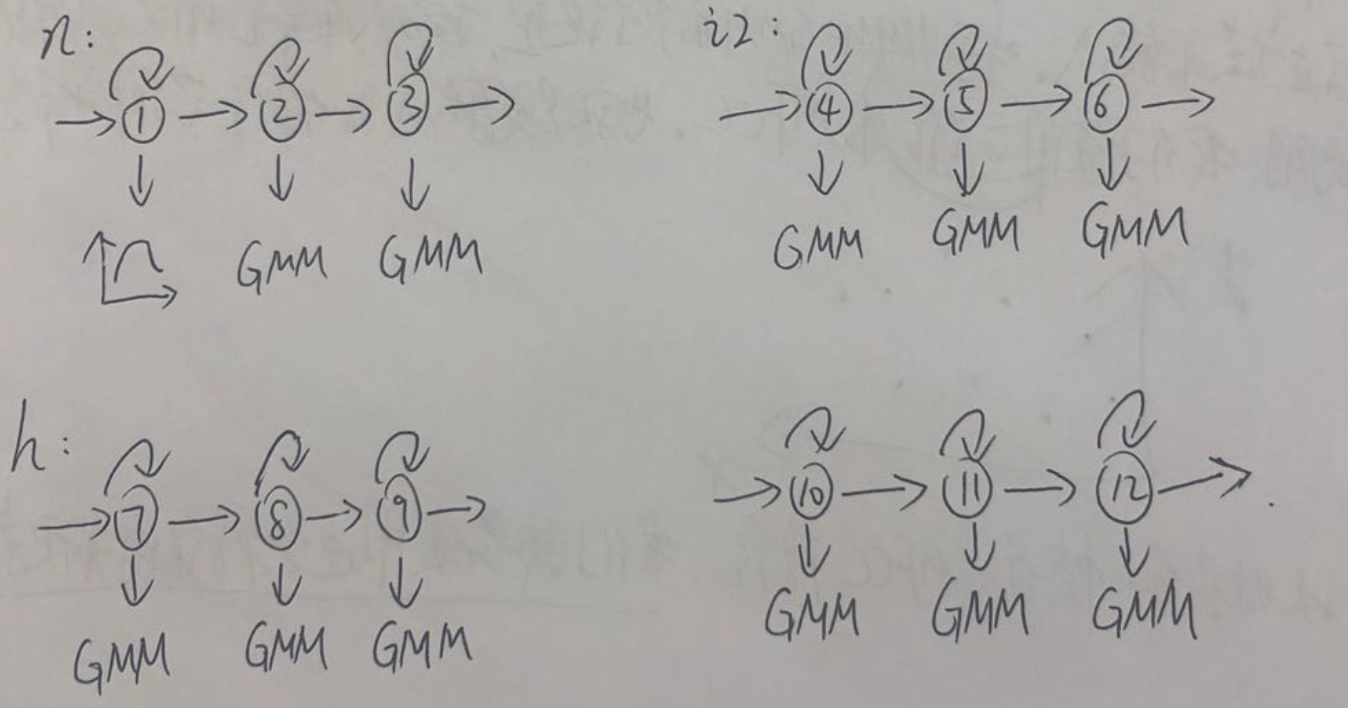###### 三、对”n“、”i2“、”h“、”ao3“对应的HMM-GMM模型进行训练，得到模型参数

• HMM-GMM模型的参数

1、转移概率
2、发射概率：因为我们使用GMM对发射概率建模，所以实际参数就是高斯分布中的均值和方差(这也是初学者容易迷糊的一个地方)。
总结：模型参数就是转移概率、高斯分布的均值、方差

• 模型的输入

输入：每帧信号的MFCC特征(也可以是fbank，plp等)。具体应用中，我们通常取39维的MFCC特征。这里为了方便说明，我们取2维的MFCC特征，也就是说输入的特征空间分布是这样的：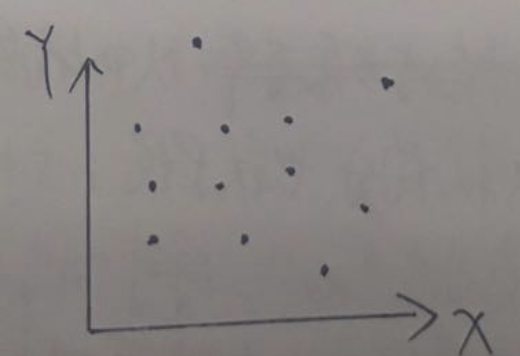上图中，每个黑点对应某一帧信号的MFCC特征。我们需要使用这些特征值来更新GMM的参数，具体怎么更新后续会讲。

• 模型的输出

输出：每一帧属于"n"、“i2”、“h”、"ao3"中的某一个状态(3状态)的概率。

• step1：初始化对齐。以上面的20秒音频为例，因为我们不知道每一帧对应哪个声韵母的某个状态，所以就均分。也就是说1-5帧对应"n"，6-10帧对应"i2"，11-15帧对应"h"，16-20帧对应"ao3"。同时"n"又有三个状态，那么就把1-2帧分给状态①，3-4帧分给状态②，第5帧分给状态③。“i2”、“h”、"ao3"亦如此。这里每个状态只是用单高斯描述，如果是混合高斯，还需要进一步用k-means算法来对每个高斯分量初始化。
初始化完成后，该段音频对应的HMM模型如下：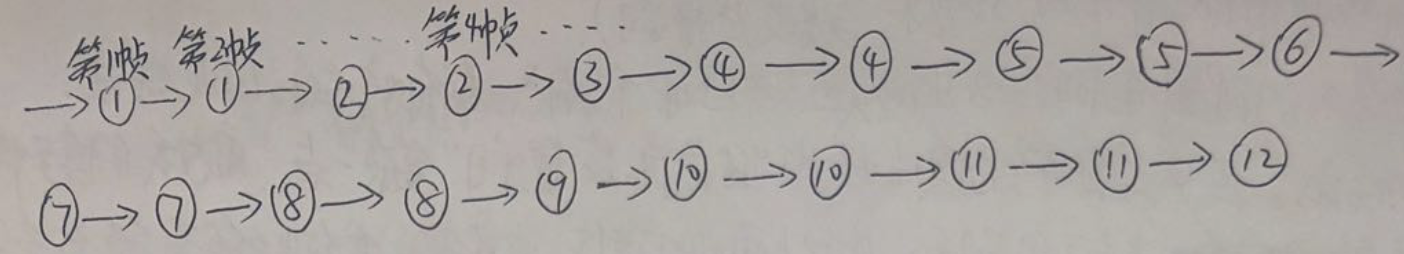• step2：更新模型参数。
1、转移概率：通过上图，我们可以得到①->①的转移次数，①->②的转移次数等等。然后除以总的转移次数，就可以得到每种转移的概率，这是一个统计的过程，无他。
2、发射概率：即均值和方差。以状态①的均值和方差为例，由上图我们可以知道第1帧和第2帧对应状态①。假设第1帧的MFCC特征是(4,3)，第2帧的MFCC特征的MFCC特征是(4,7)。那么状态①的均值就是(4,5)，方差是(0,8)

• step3：重新对齐。根据step2得到的参数，重新对音频进行状态级别的对齐。这一步区别于step1的初始化，step1的初始化我们是采用粗暴的均匀对齐，而这一步的对齐是根据step2的参数进行对齐的。这里的对齐方法有两种：a、硬对齐：采用维特比算法；b、软对齐：采用前后向算法。
经过重新对齐后，可能变成这样(第2帧已经不对应状态①了)：• step4：重复step2和step3多次，直到收敛。

2018-11-06 05:22:38 kahncc 阅读数 182
• ###### C++语音识别开篇

本篇mark老师将教大家使用第三方库的调用来简单的实现语音识别。随着机器学习和人工智能的热闹，国内语音行业也可谓是百花齐放。 语音识别一个伟大的时代已在我们身边悄悄走来。

5918 人正在学习 去看看 杨波

1. 什么是Hidden Markov Model

HMM要解决的三个问题:

1) Likelihood

2) Decoding

3) Training

2. GMM是神马？怎样用GMM求某一音素（phoneme）的概率？

3. GMM+HMM大法解决语音识别

3.1 识别

3.2 训练

3.2.1 Training the params of GMM

3.2.2 Training the params of HMM

====================================================================

1. 什么是Hidden Markov Model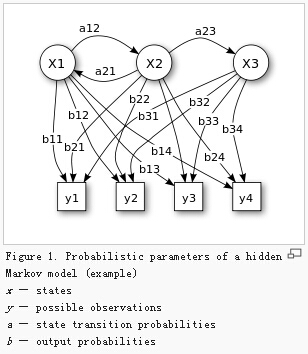ANS：一个有隐节点（unobservable）和可见节点（visible）的马尔科夫过程（见详解）。

1. N个状态的初始概率

2. 状态转移概率a

3. 输出概率b

--------------

• 在语音处理中，一个word由若干phoneme（音素）组成；
• 每个HMM对应于一个word或者音素（phoneme）
• 一个word表示成若干states，每个state表示为一个音素

1）. Likelihood: 一个HMM生成一串observation序列x的概率< the Forward algorithm>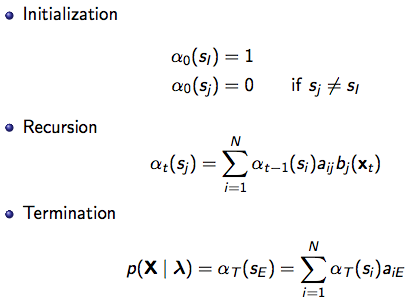aij是状态i到状态j的转移概率，

bj（xt）表示在状态j的时候生成xt的概率，

2）. Decoding: 给定一串observation序列x，找出最可能从属的HMM状态序列< the Viterbi algorithm>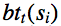为：从时刻t-1的哪个状态转移到时刻t时状态为j的概率最大；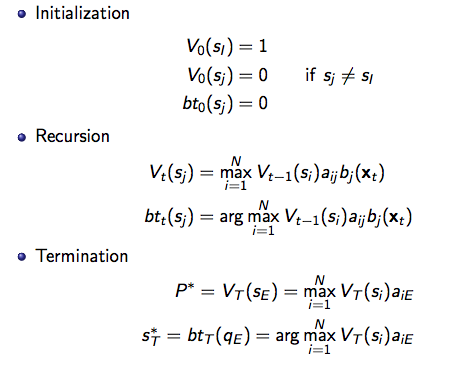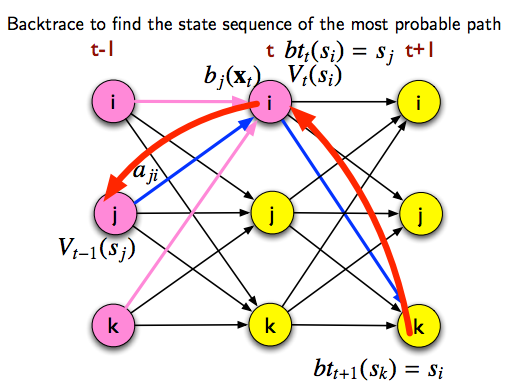3）. Training: 给定一个observation序列x，训练出HMM参数λ = {aij, bij}  the EM (Forward-Backward) algorithm

---------------------------------------------------------------------

2. GMM是神马？怎样用GMM求某一音素（phoneme）的概率？

2.1 简单理解混合高斯模型就是几个高斯的叠加。。。e.g. k=3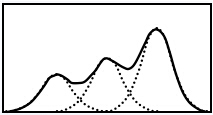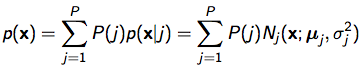fig2. GMM illustration and the probability of x

2.2 GMM for state sequence

PS：sil表示silence（静音）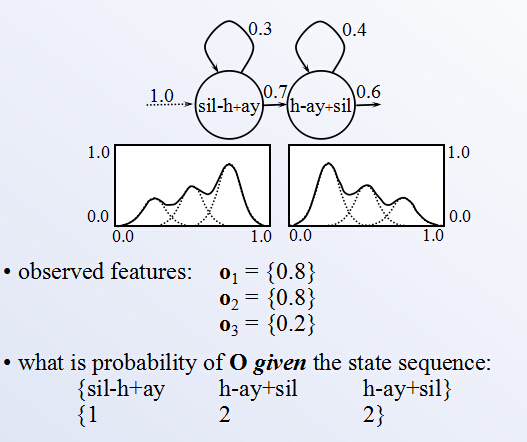fig3. use GMM to estimate the probability of a state sequence given observation {o1, o2, o3}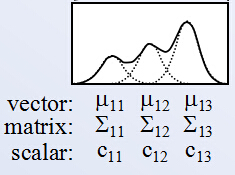fig4. parameters of a GMM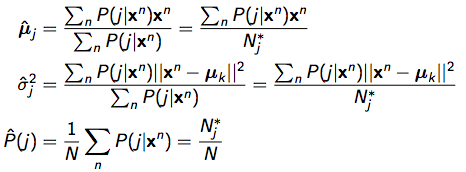， 其中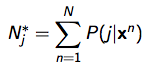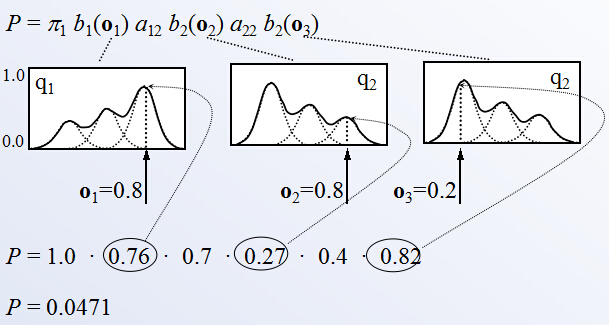fig5. probability of S1->S2->S3 given o1->o2->o3

---------------------------------------------------------------------

3. GMM+HMM大法解决语音识别

<!--识别-->

1). 将waveform切成等长frames，对每个frame提取特征（e.g. MFCC）,

2).对每个frame的特征跑GMM，得到每个frame(o_i)属于每个状态的概率b_state(o_i)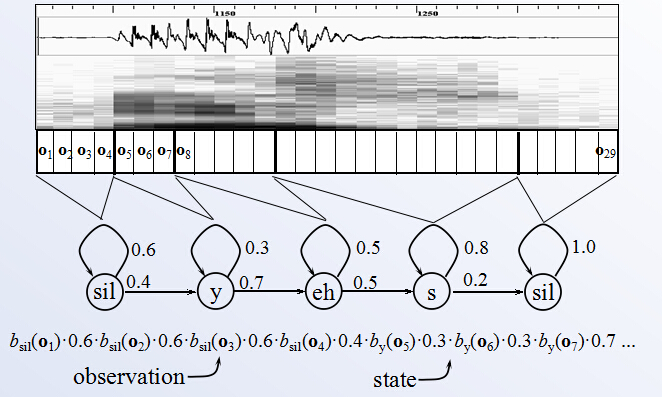fig6. complete process from speech frames to a state sequence

3). 根据每个单词的HMM状态转移概率a计算每个状态sequence生成该frame的概率; 哪个词的HMM 序列跑出来概率最大，就判断这段语音属于该词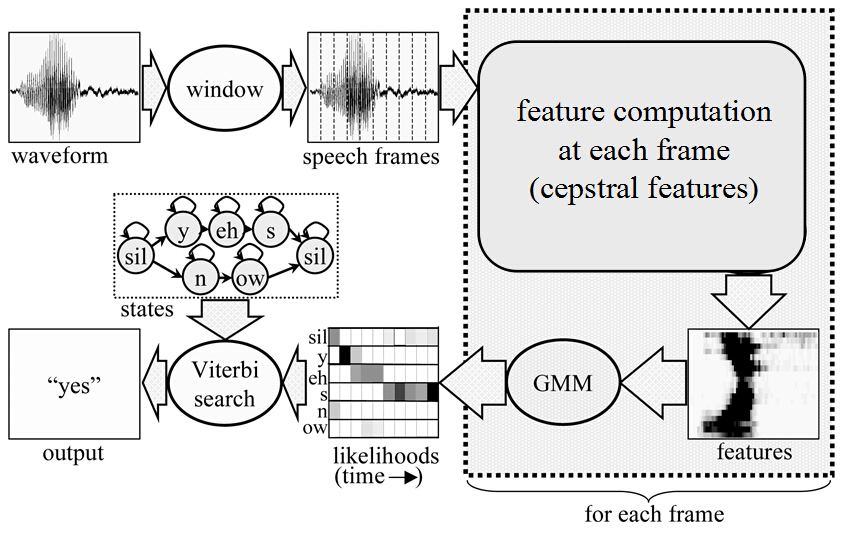fig7. Speech recognition, a big framework

(from Encyclopedia of Information Systems, 2002)

<!--训练-->

①Training the params of GMM

GMM参数：高斯分布参数：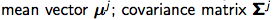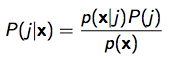fig8. bayesian formula of P( j | x )

A. 初始化（可以用kmeans）得到P(j)

B. 迭代

E（estimate）-step: 根据当前参数 (means, variances, mixing parameters)估计P(j|x)

M（maximization）-step: 根据当前P(j|x) 计算GMM参数（根据fig4 下面的公式：）， 其中②Training the params of HMM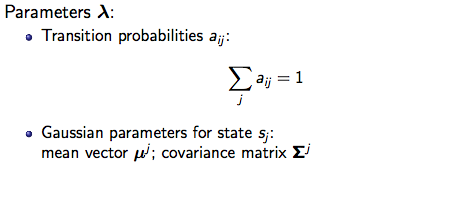HMM训练过程：迭代

E（estimate）-step: 给定observation序列，估计时刻t处于状态sj的概率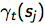M（maximization）-step: 根据重新估计HMM参数aij.

E-step: 给定observation序列，估计时刻t处于状态sj的概率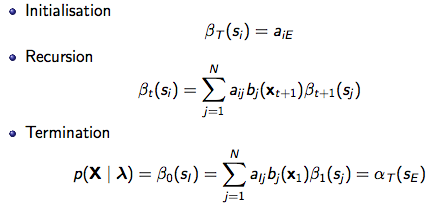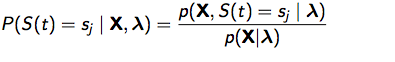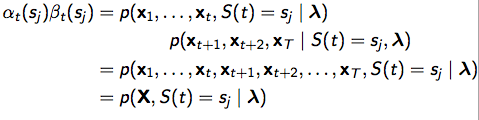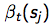: t时刻处于状态sj的话，t时刻未来observation的概率；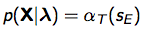finally, 带入的定义式有：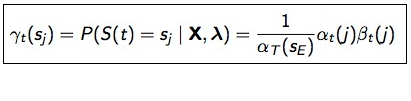M-step：根据重新估计HMM参数λ：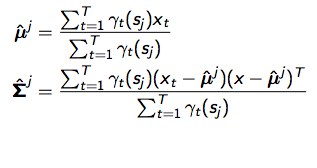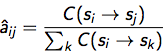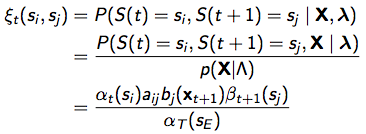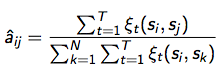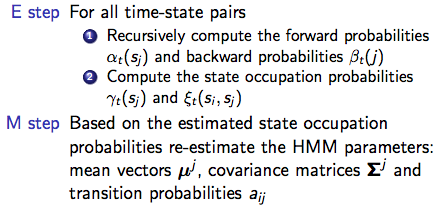PS：这个训练HMM的算法叫 Forward-Backward algorithm。

#### 给我老师的人工智能教程打call！http://blog.csdn.net/jiangjunshow